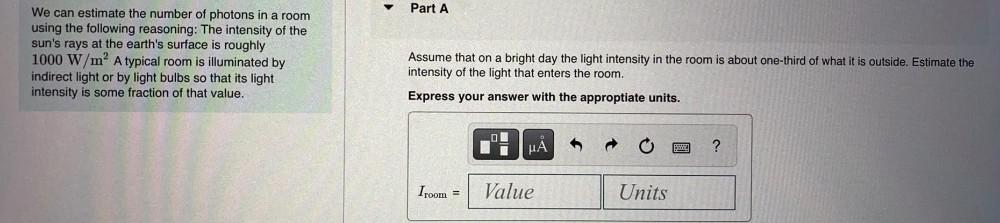Question:

# Part A We can estimate the number of photons in a room using the following reasoning: The intensity of the sun's rays at the earPart A We can estimate the number of photons in a room using the following reasoning: The intensity of the sun's rays at the earth's surface is roughly 1000 W/m? A typical room is illuminated by indirect light or by light bulbs so that its light intensity is some fraction of that value. Assume that on a bright day the light intensity in the room is about one-third of what it is outside. Estimate the intensity of the light that enters the room. Express your answer with the approptiate units. uả pe ? Iroom = Value Units